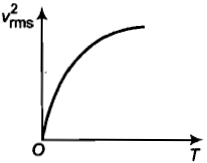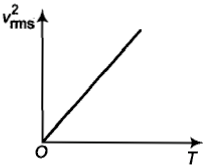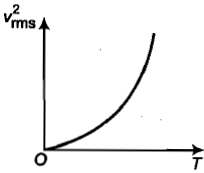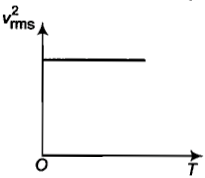Choose the correct option related to the specific heat at constant volume ${C}_{V}$ -

(1) ${C}_{V}=\frac{1}{2}fR$              (2) ${C}_{V}=\frac{R}{\gamma -1}$

(3) ${C}_{V}=\frac{{C}_{P}}{\gamma }$                (4) All of these

Concept Questions :-

Specific heat
High Yielding Test Series + Question Bank - NEET 2020

Difficulty Level:

The curve between absolute temperature and ${{v}_{rms}}^{2}$ is:

(a)(b)(c)(d)Concept Questions :-

Types of velocity
High Yielding Test Series + Question Bank - NEET 2020

Difficulty Level:

Five molecules of a gas have speeds, 1, 2, 3, 4 and 5 km${s}^{-1}$.  The value of the root mean square speed of the gas molecules is:

(1) 3 km${s}^{-1}$             (2) $\sqrt{11}$km${s}^{-1}$

(3) $\frac{1}{2}\sqrt{\mathrm{\pi }}$km${s}^{-1}$    (4) 3.5 km${s}^{-1}$

Concept Questions :-

Types of velocity
High Yielding Test Series + Question Bank - NEET 2020

Difficulty Level:

The average velocity of an ideal gas molecule is:                                                                                                                   [OJEE 2010]

(1) proportional to $\sqrt{T}$

(2) proportional to ${T}^{2}$

(3) proportional to ${T}^{3}$

(4) zero

Concept Questions :-

Types of velocity
High Yielding Test Series + Question Bank - NEET 2020

Difficulty Level:

For a gas, $\frac{R}{{C}_{V}}=0.67$.  This gas is made up of molecules which are:                                                                   [JIPMER 2001, 02]

(1) Diatomic           (2) Mixture of diatomic and polyatomic molecules

(3) Monoatomic      (4) Polyatomic

Concept Questions :-

Specific heat
High Yielding Test Series + Question Bank - NEET 2020

Difficulty Level:

At constant temperature, on increasing the pressure of a gas by 5% its volume will decrease by:          [MP PET 2002; Kerala 2006]

(1) 5%       (2) 5.26 %         (3) 4.26 %         (4) 4.76 %

Concept Questions :-

Ideal gas
High Yielding Test Series + Question Bank - NEET 2020

Difficulty Level:

Concept Questions :-

Types of velocity
High Yielding Test Series + Question Bank - NEET 2020

Difficulty Level:

At what temperature, is the root mean square velocity of gaseous hydrogen molecules equal to that of oxygen molecules at 47$°$C:[MP PET 1997, 2000; RPET 1999; AIEEE 2002; J & K CET 2004; Kerala PET 2010]

(1) 20 K           (2) 80 K           (3) - 73 K              (4) 3 K

Concept Questions :-

Types of velocity
High Yielding Test Series + Question Bank - NEET 2020

Difficulty Level:

The average kinetic energy of a gas molecule can be determined by knowing:                                        [RPET 2000, MP PET 2010]

(1) The number of molecules in the gas

(2) The pressure of the gas only

(3) The temperature of the gas only

(4) None  of the above is enough by itself

Concept Questions :-

Kinetic energy of gas
High Yielding Test Series + Question Bank - NEET 2020

Difficulty Level:

Volume, pressure, and temperature of an ideal gas are V, P, and T respectively. If the mass of its molecule is m, then its density is [k=boltzmann's constant]

(a) mkT                              (b) $\frac{P}{kT}$

(d)$\frac{P}{kTV}$                             (d) $\frac{Pm}{kT}$

Concept Questions :-

Ideal gas